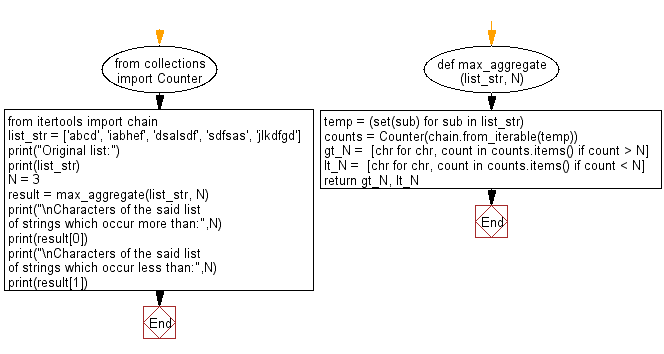﻿ Python: Find the characters which occur in more than and less than a given number in list of strings - w3resource# Python: Find the characters which occur in more than and less than a given number in list of strings

## Python Collections: Exercise-25 with Solution

Write a Python program to find the characters in a list of strings which occur more than and less than a given number.

Sample Solution:

Python Code:

``````from collections import Counter
from itertools import chain
def max_aggregate(list_str, N):
temp = (set(sub) for sub in list_str)
counts = Counter(chain.from_iterable(temp))
gt_N =  [chr for chr, count in counts.items() if count > N]
lt_N =  [chr for chr, count in counts.items() if count < N]
return gt_N, lt_N

list_str = ['abcd', 'iabhef', 'dsalsdf', 'sdfsas', 'jlkdfgd']
print("Original list:")
print(list_str)
N = 3
result = max_aggregate(list_str, N)
print("\nCharacters of the said list of strings which occur more than:",N)
print(result)
print("\nCharacters of the said list of strings which occur less than:",N)
print(result)
```
```

Sample Output:

```Original list:
['abcd', 'iabhef', 'dsalsdf', 'sdfsas', 'jlkdfgd']

Characters of the said list of strings which occur more than: 3
['a', 'd', 'f']

Characters of the said list of strings which occur less than: 3
['c', 'b', 'h', 'e', 'i', 's', 'l', 'k', 'j', 'g']
```

Flowchart:## Visualize Python code execution:

The following tool visualize what the computer is doing step-by-step as it executes the said program:

Python Code Editor:

Have another way to solve this solution? Contribute your code (and comments) through Disqus.

What is the difficulty level of this exercise?

Test your Programming skills with w3resource's quiz.

﻿

## Python: Tips of the Day

The Zip() Function:

```>>> students = ('John', 'Mary', 'Mike')
>>> ages = (15, 17, 16)
>>> scores = (90, 88, 82, 17, 14)
>>> for student, age, score in zip(students, ages, scores):
...     print(f'{student}, age: {age}, score: {score}')
...
John, age: 15, score: 90
Mary, age: 17, score: 88
Mike, age: 16, score: 82
>>> zipped = zip(students, ages, scores)
>>> a, b, c = zip(*zipped)
>>> print(b)
(15, 17, 16)
```